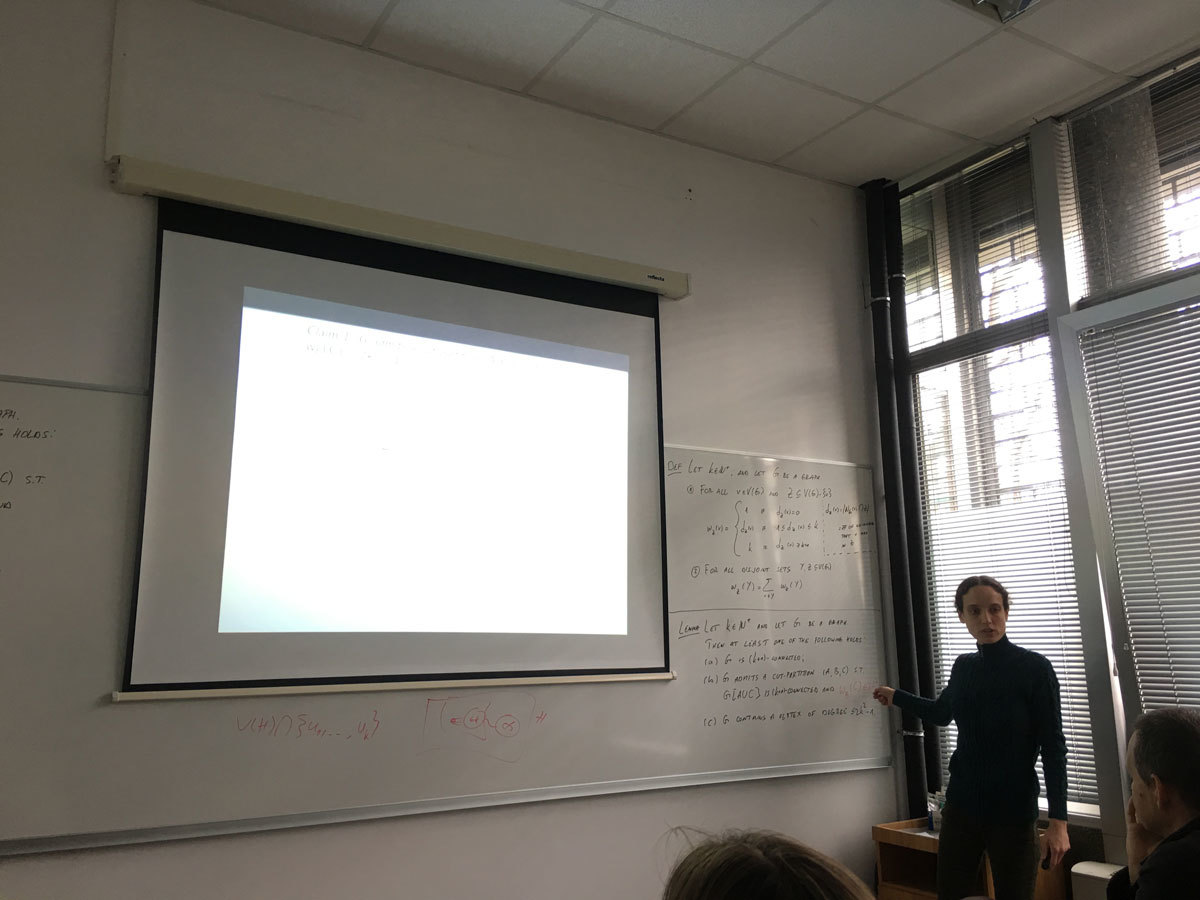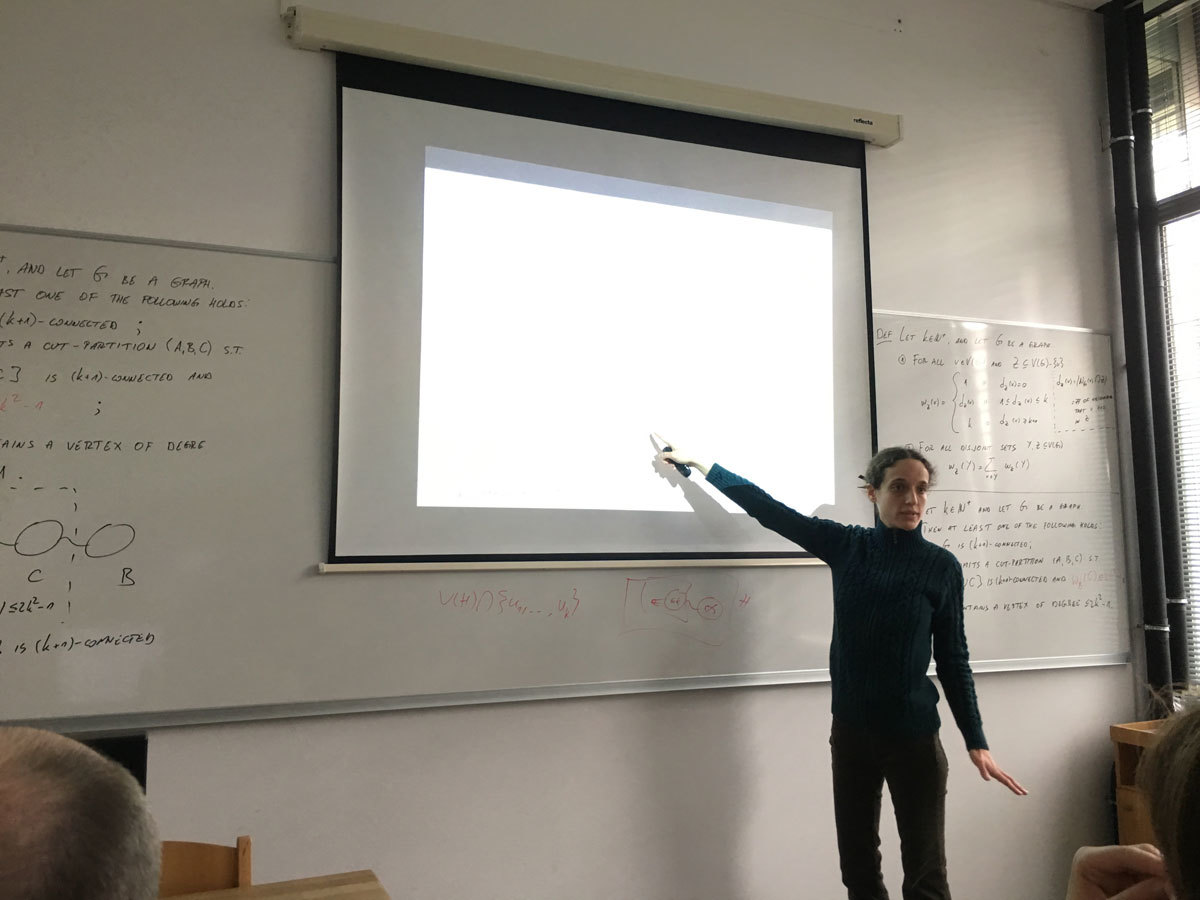Univerza na Primorskem Fakulteta za matematiko, naravoslovje in informacijske tehnologije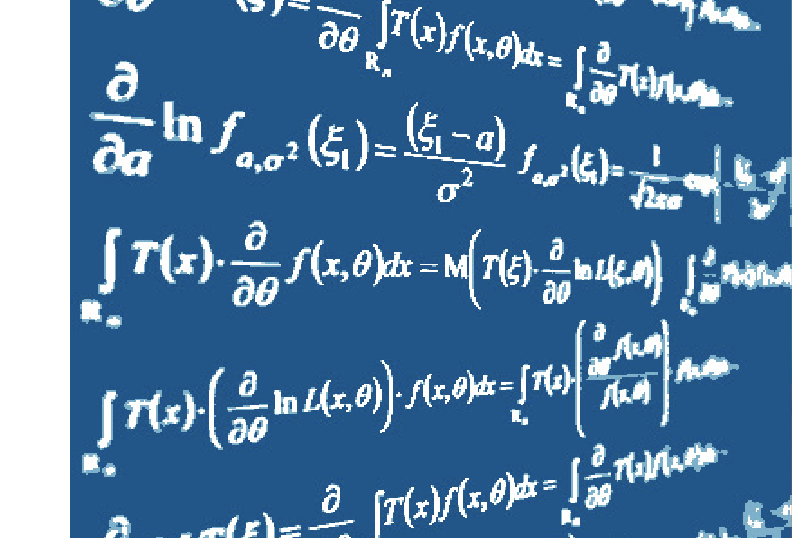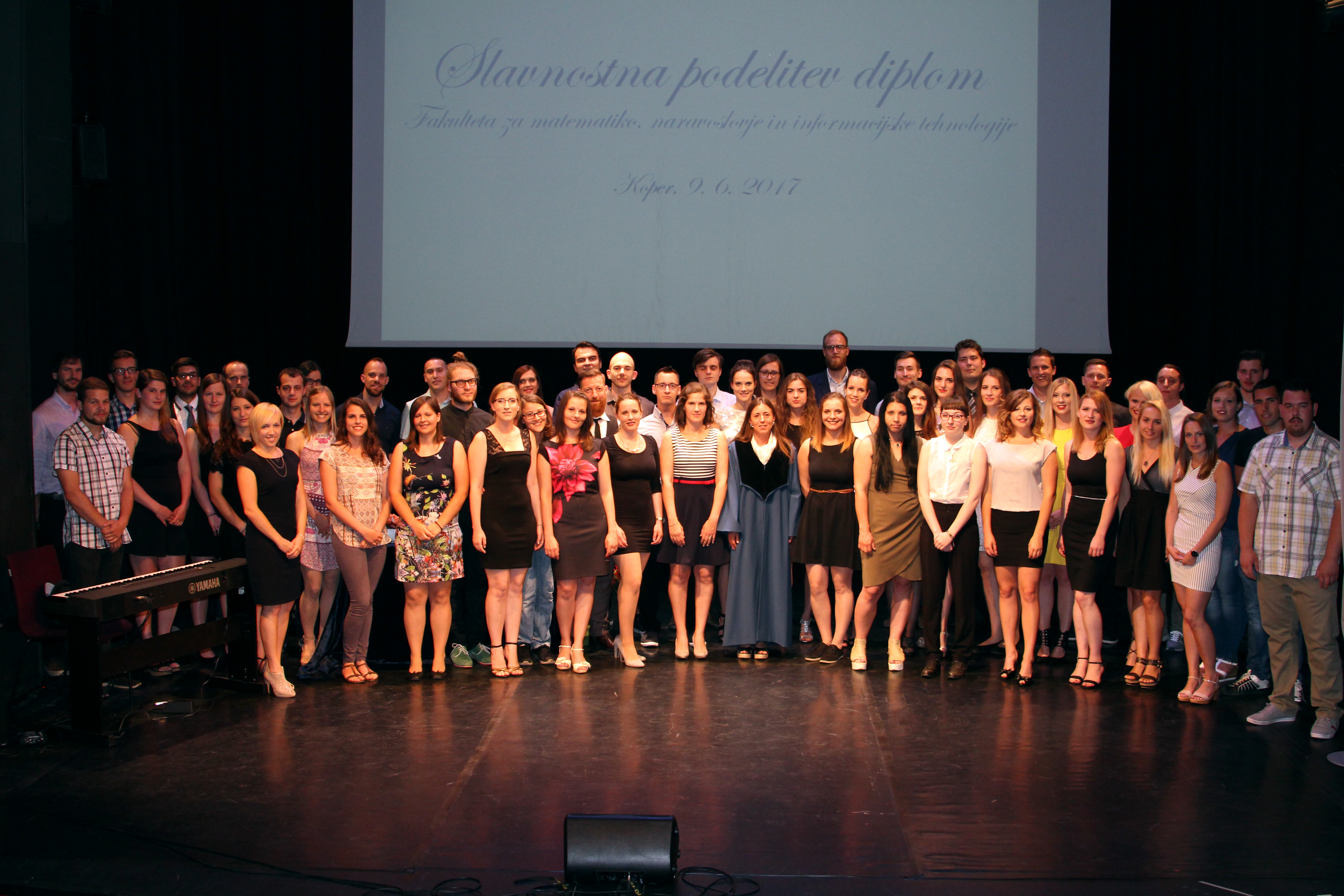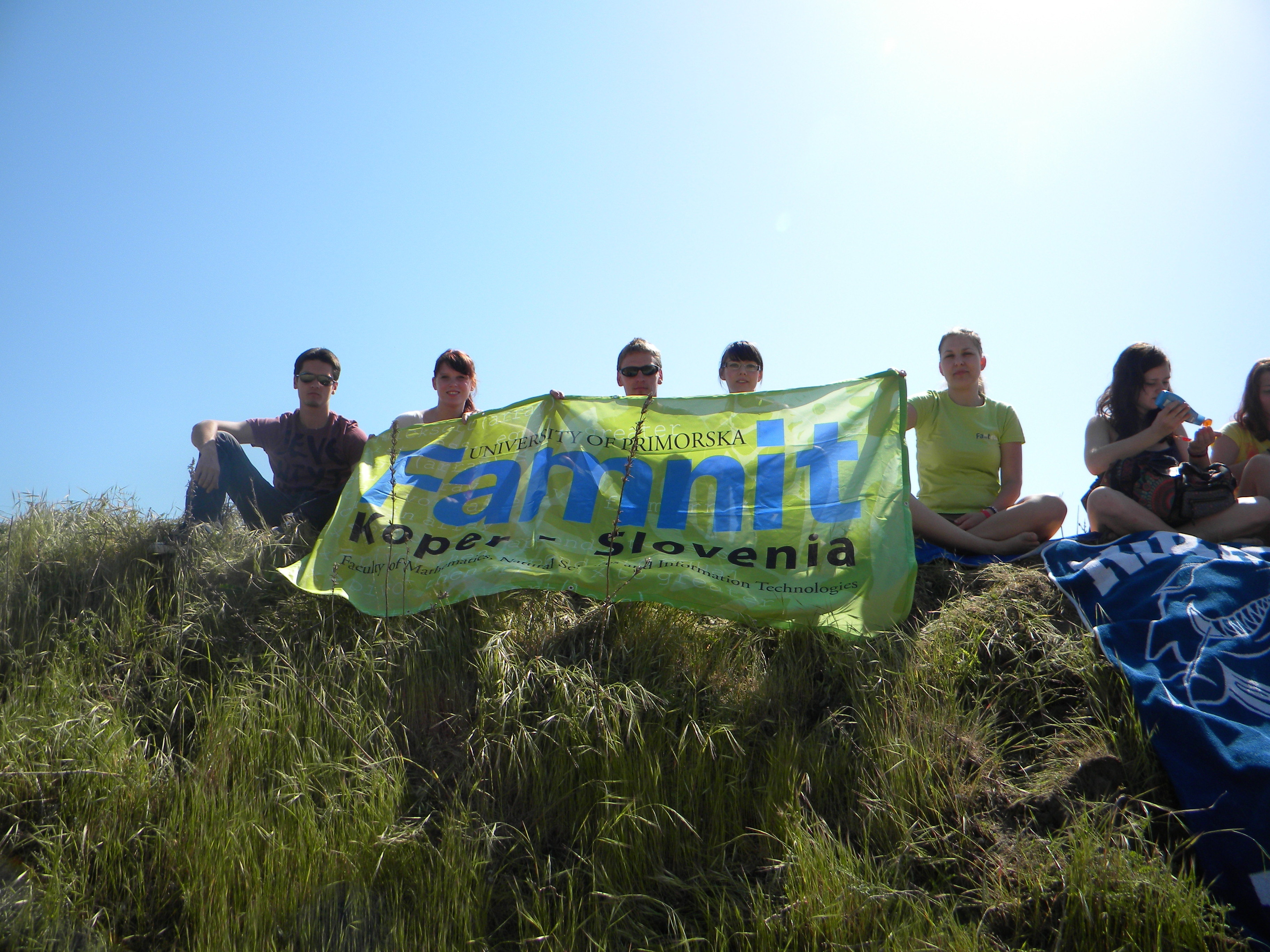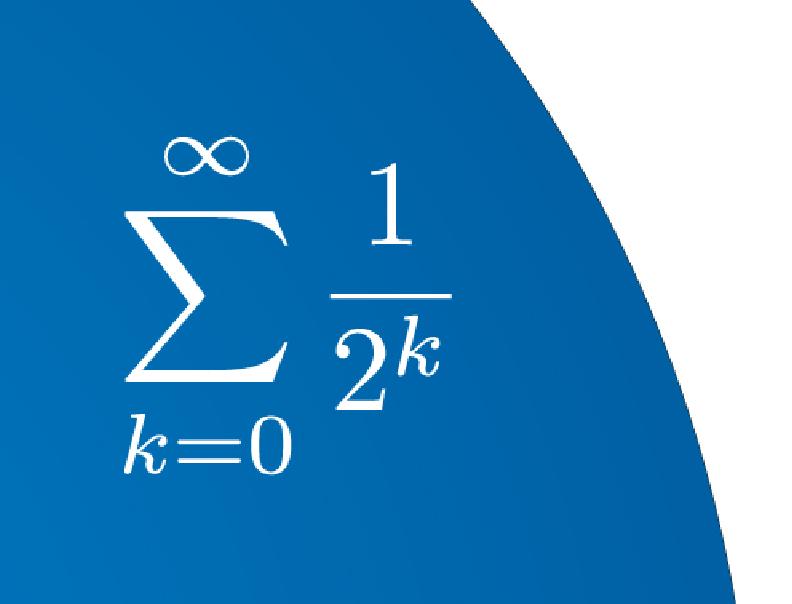SI | EN# Raziskovalni matematični seminar - Arhiv

 2021 2020 2019 2018 2017 2016 2015 2014 2013 2012 2011 2010
 1 2 3 4 5 6 7 8 9 10 11 12
Datum in ura / Date and time: 31.1.18
(10:00-11:00)
Predavalnica / Location: FAMNIT-POŠTA
Predavatelj / Lecturer: Monica Milasi (University of Messina)
Naslov / Title: Generalized convexity and applications.
Vsebina / Abstract:

The aim of this talk is to consider the quasiconvex and pseudoconvex functions. One of the reasons for the study of generalized convexity is that convexity usually is a rather rigid assumption, and often it is not satisfied in real-world applications. Instead, the generalized convex functions mantain good properties and it are used in several areas of science, including scalar and vector optimization, operations research, mathematical economics.

After introducing this class of functions, we will deal with  the role of the generalized convexity in Optimization and in the economic applications.

Lectures will be held:
Wednesday 31st of January 2018. from 10:00 -- 11:00 (FAMNIT-POŠTA)
Friday 2nd of February 2018. from 10:00 -- 12:00 (FAMNIT-POŠTA)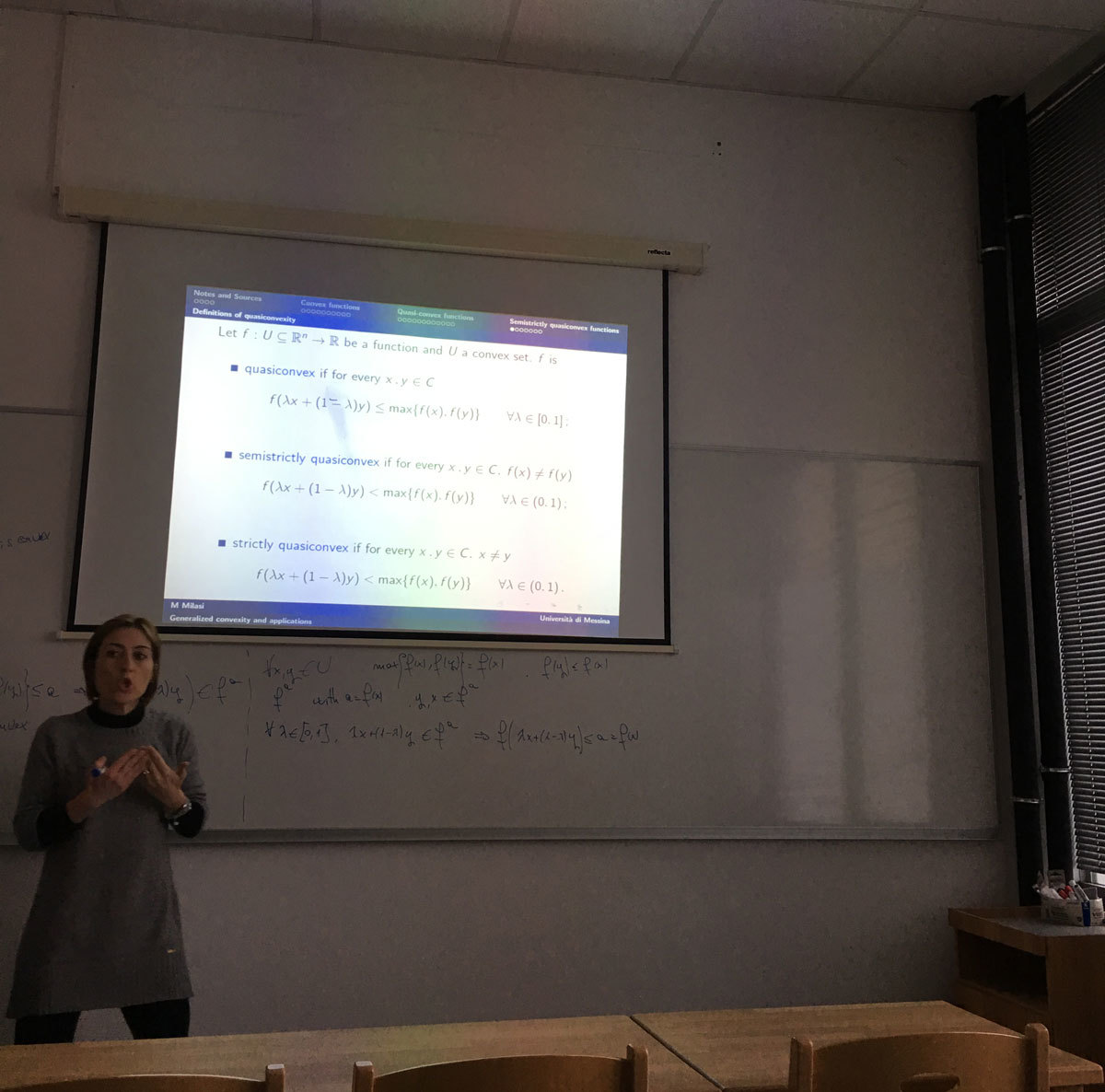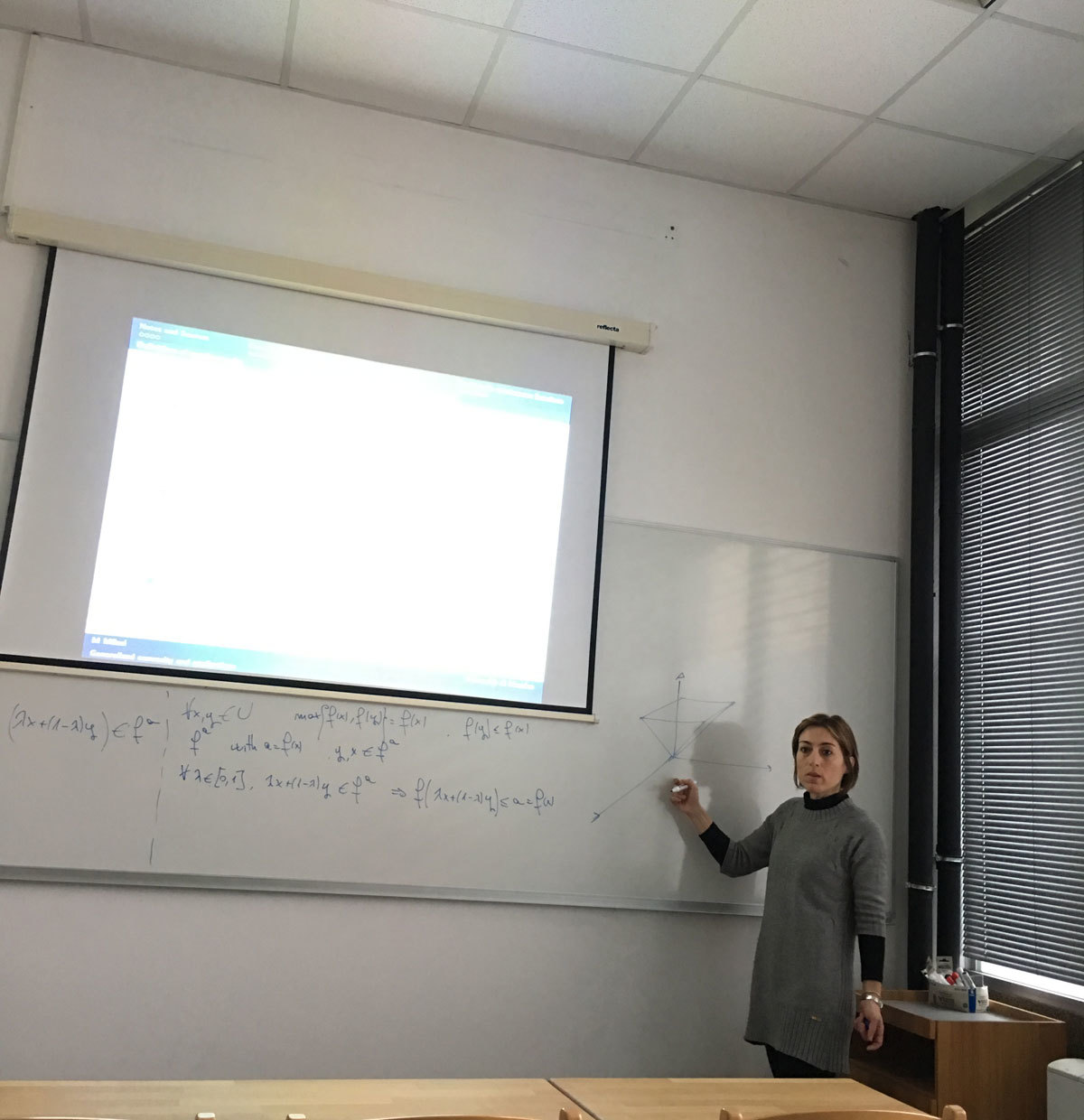Datum in ura / Date and time: 24.1.18
(11:00-12:30)
Predavalnica / Location: FAMNIT-Muzejski1
Predavatelj / Lecturer: Mikhail E. Muzychuk (Ben-Gurion University of the Negev, Israel)
Naslov / Title: Coherent configurations
Vsebina / Abstract:

In my lectures I'll talk about coherent configurations, association schemes and their connection to combinatorics and finite geometry. We also learn how coherent configurations are related to the Graph Isomorphism Problem. Also a brief introduction to the Weisfeiler-Leman algorithm will be given.

Lectures will be:
Wednesday 24th of January 2018. from 11:00 -- 12:30 (FAMNIT-Muzejski1)
Friday 26th of January 2018. from 11:00 -- 12:30 (FAMNIT-Muzejski1)
Monday 29th of January 2018 from 11:00 -- 12:30 (FAMNIT-Pošta)

Datum in ura / Date and time: 22.1.18
(10:00-11:00)
Predavalnica / Location: FAMNIT-POŠTA
Predavatelj / Lecturer: Alejandra Ramos (UP IAM & UP FAMNIT)
Naslov / Title: Structural results on vertex- and edge-transitive graphs
Vsebina / Abstract:

In this talk we present the main topics, research questions and the expected results of the proposed PhD thesis.

The central theme of the PhD thesis are graphs admitting a considerable degree of symmetry. More precisely, we focus on graphs admitting a vertex- and edge-transitive group of automorphisms.

A graph Γ is said to be G-vertex-transitive, G-edge-transitive and G-arc-transitive whenever the subgroup G ≤ Aut(Γ) acts transitively on V(Γ), E(Γ) and A(Γ), respectively. We say that Γ is G-half-arc-transitive (abbreviated by G-HAT) if it is G-vertex- and G-edge-transitive but not G-arc-transitive. In the case of G=Aut(Γ), we omit the prefix G and simply write vertex-transitive, edge-transitive, arc-transitive and half-arc-transitive (abbreviated by HAT).

Let Γ be a G-vertex- and G-edge-transitive graph for some G ≤ Aut(Γ). Then two essentially different possibilities can occur:
(i) Γ is G-arc-transitive.
(ii) Γ is G-half-arc-transitive.

In the first main topic of the PhD thesis we will focus on the situations from the above possibility (i). In particular, we will be interested in the application of the properties of arc-transitive graphs as a tool in the investigation of symmetries of maps.

In the second and third part of the PhD thesis, we will focus on the situations from the above possibility (ii). We plan to introduce a new parameter of tetravalent G-HAT graphs, giving a better understanding of the structural properties of such graphs. We will study the properties of the graphs with respect to this parameter and use it to relate two important approaches for a possible classification of all tetravalent G-HAT graphs. We will also improve the results on the question of whether the attachment number divides the radius for all tetravalent HAT graphs.

Finally, we will focus on HAT graphs with valencies greater than four. We will generalize the Bouwer graphs to obtain a much larger family of vertex- and edge-transitive graphs, most of whose members are in fact tightly attached HAT graphs.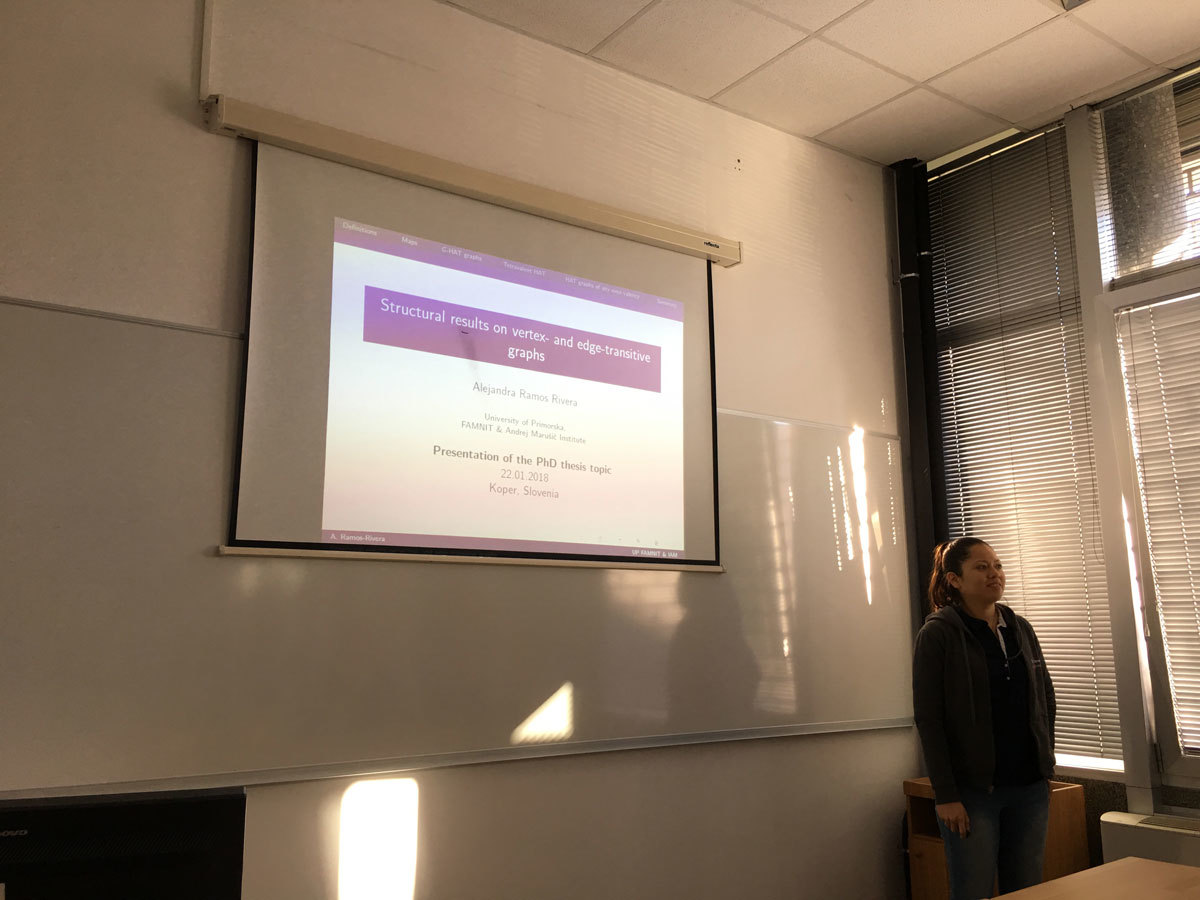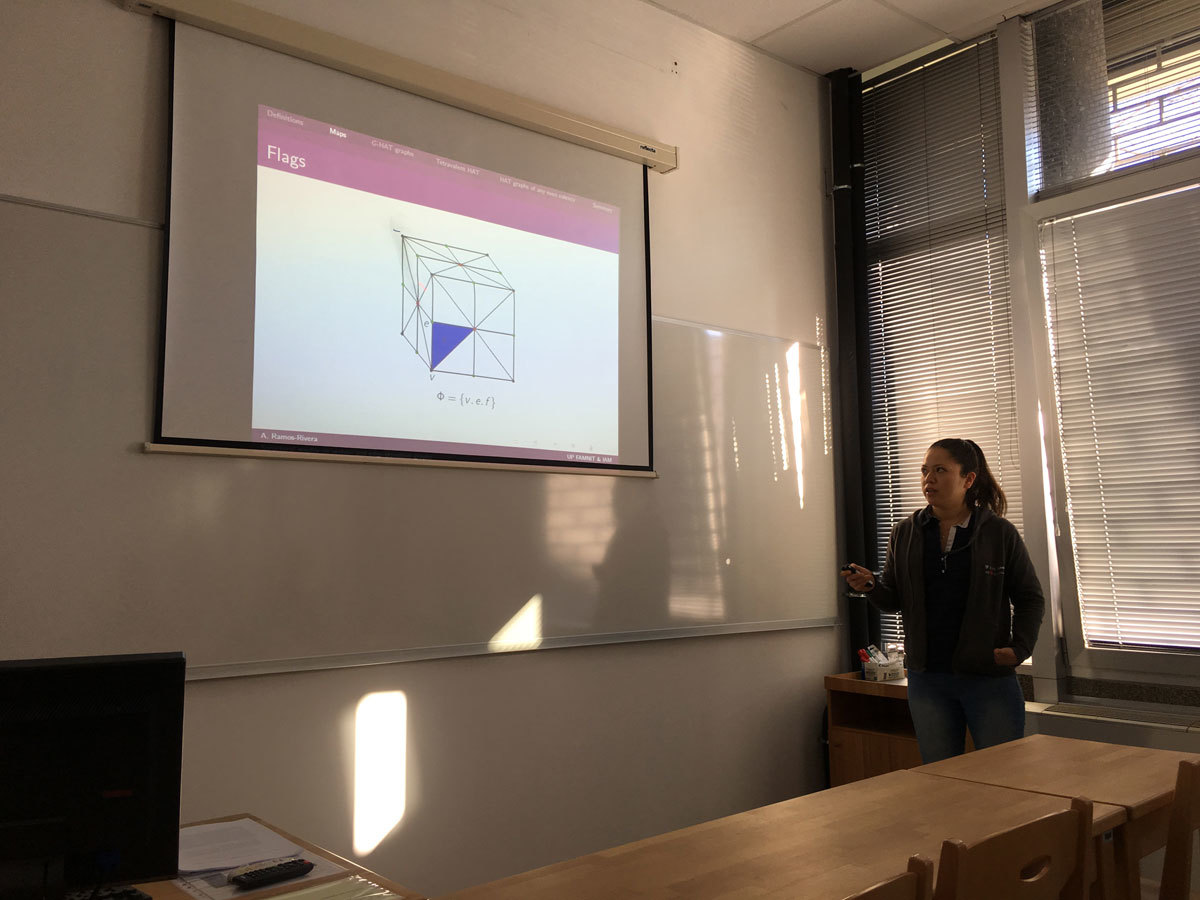Datum in ura / Date and time: 15.1.18
(10:00-11:00)
Predavalnica / Location: FAMNIT-POŠTA
Predavatelj / Lecturer: Bojan Kuzma (UP FAMNIT)
Naslov / Title: Commuting graphs -- Isometry transfer problems, realizability questions, property recognition, and homomorphisms
Vsebina / Abstract:

The essence of commutativity relation on a given Magma is captured in its commuting graph which, by definition, is a simple graph on noncentral elements as vertices and where two distinct vertices a,b form an edge if ab=ba. The commuting graph can be an important tool in investigation of nonabelian Magmas which lack the notion of a commutator (say, in semigroups) but can also be studied in nonabelian groups or algebras.

In the talk we aim to show a few examples of isometry transfer problems, i.e., that in certain class of (semi)groups and matrix algebras the commuting graphs are isomorphic if and only if the corresponding algebraic sets are isomorphic. We will also consider realizability questions, i.e., which graph can be obtained as a commuting graph of a semigroup or of a group.

Time permitting, we will further address property recognition for matrix algebras, i.e., how to determine from commuting graph if a matrix has, say, rank-one or is diagonalizable, and/or review basic ideas in classification of homomorphisms of certain commuting (and anti-commuting) graphs.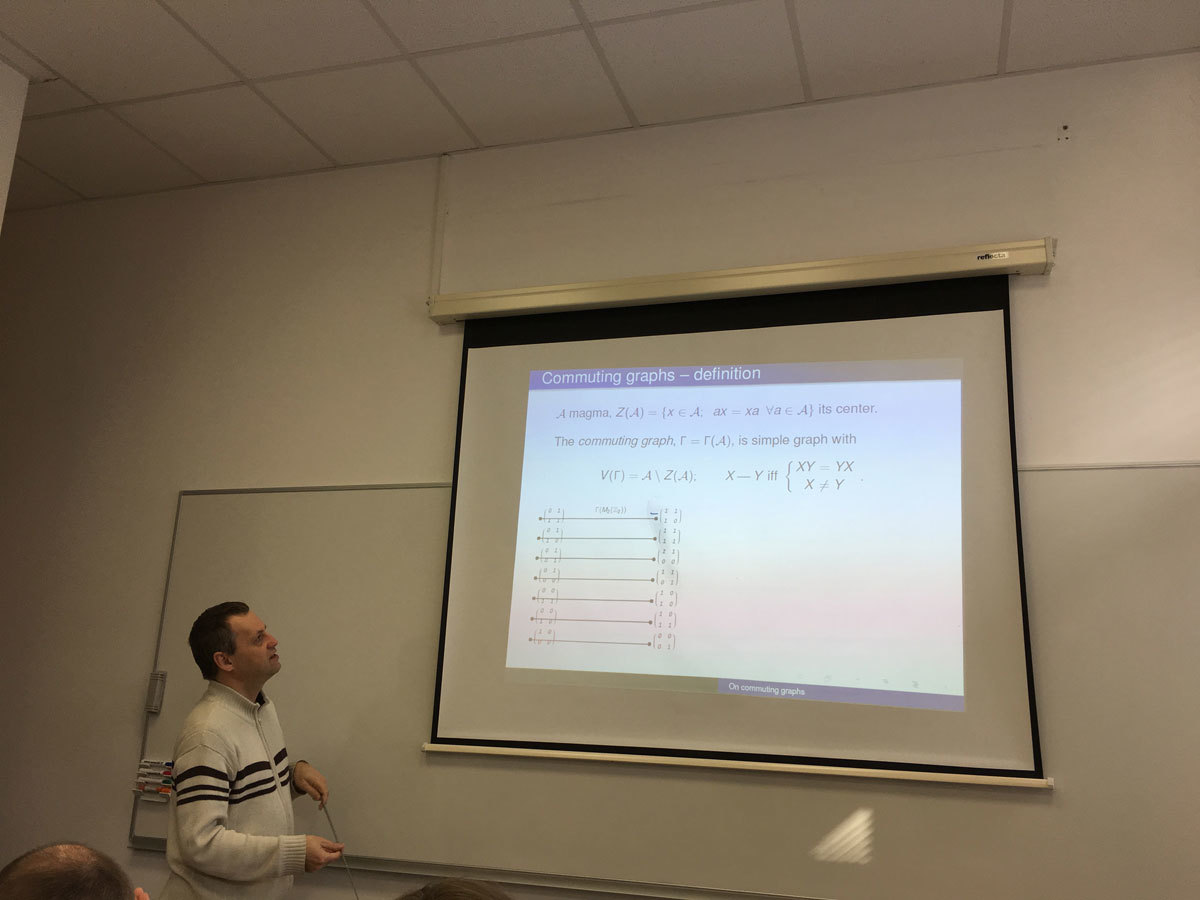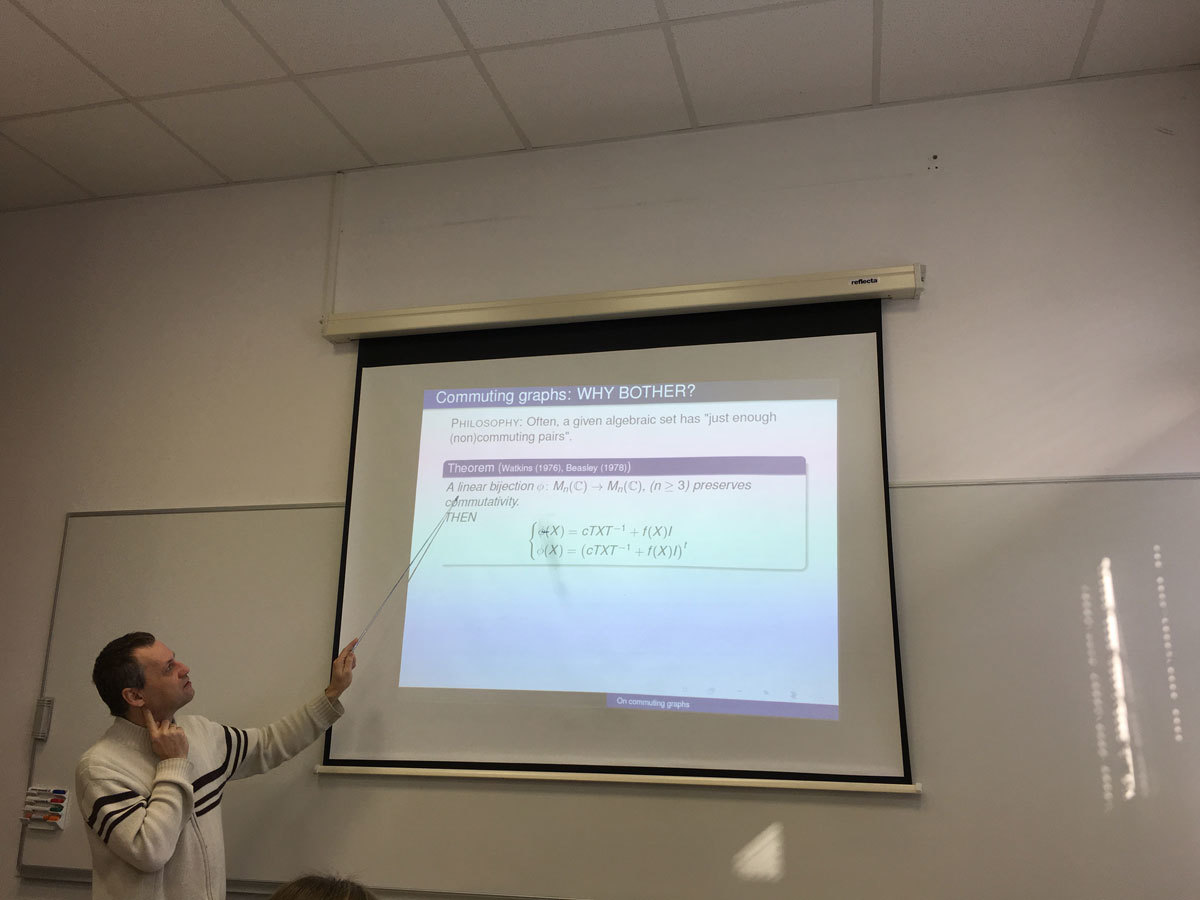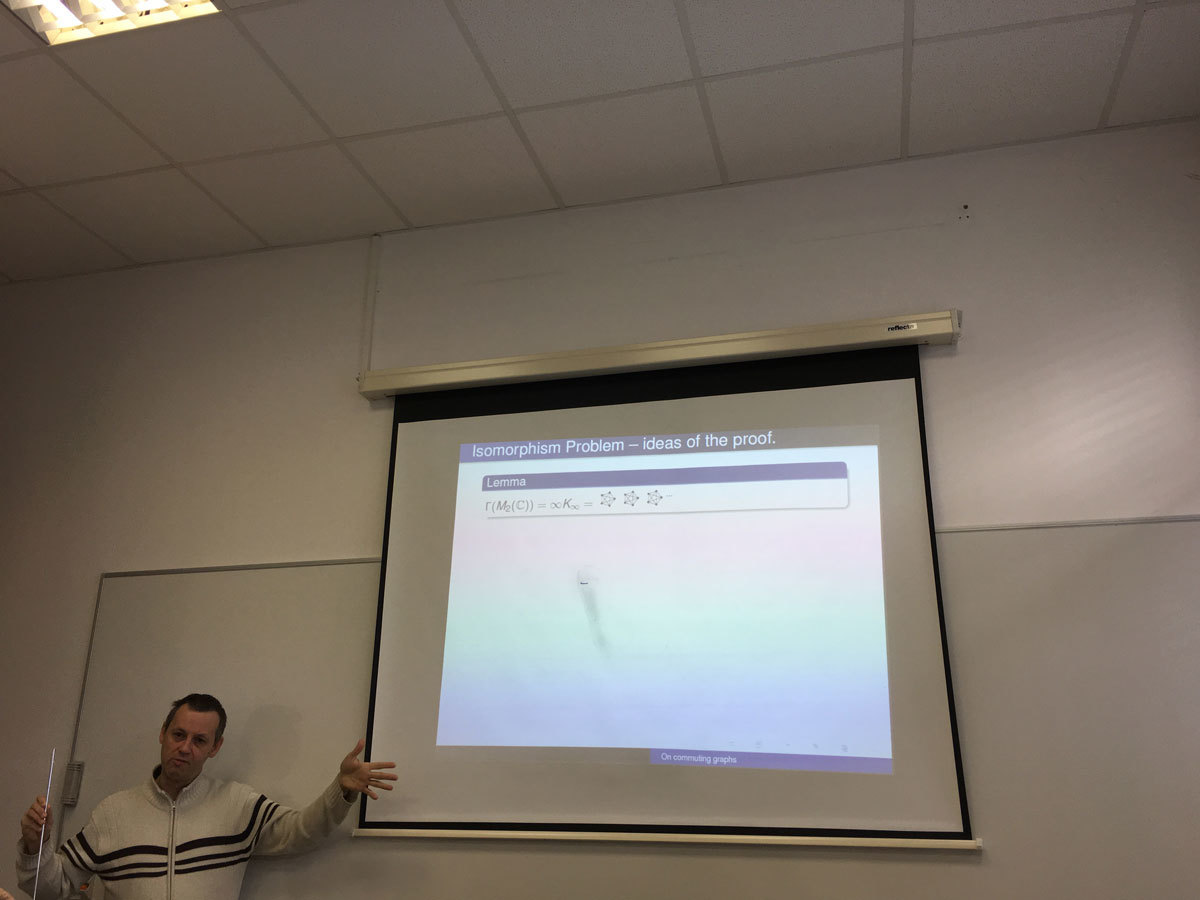Datum in ura / Date and time: 8.1.18
(10:00-11:00)
Predavalnica / Location: FAMNIT-POŠTA
Predavatelj / Lecturer: Irena Penev (University of Leeds, United Kingdom)
Naslov / Title: Isolating highly connected induced subgraphs
Vsebina / Abstract:

Mader (1972) proved that every graph of average degree at least 4k contains a (k+1)-connected subgraph, and Alon, Kleitman, Saks, Seymour, and Thomassen (1987) proved that every graph of chromatic number greater than \max\{c+10k^2,100k^3\} contains a (k+1)-connected subgraph of chromatic number at least c. We prove a variant of Mader's theorem (with stronger hypotheses and a stronger conclusion), and we improve the bound in the theorem of Alon et al.

Given a graph G, \delta(G) is the minimum degree of G. If H is an induced subgraph of G, then \partial_G(H) is the set of all vertices of H that have a neighbor in V(G) \setminus V(H). Our main results are the following two theorems.

Theorem 1. Let k be a positive integer, and let G be a graph. If \delta_G(G) > 2k^2-1, then G contains a (k+1)-connected induced subgraph H such that \partial_G(H) \subsetneqq V(H) and |\partial_G(H)| \leq 2k^2-1.

Theorem 2. Let k and c be positive integers, and let G be a graph such that \chi(G) > \max\{c+2k-2,2k^2\}. Then G contains a (k+1)-connected induced subgraph of chromatic number greater than c.

A {\em cut-partition} of a graph G is a partition (A,B,C) of V(G) such that A and B are non-empty (C may possibly be empty), and there are no edges between A and B. Theorem 1 is an immediate corollary of a certain technical lemma, which roughly states that if the minimum degree of a graph G is greater than 2k^2-1, then either G is (k+1)-connected, or G admits a cut-partition (A,B,C) such that G[A \cup C] is (k+1)-connected, |C| \leq 2k^2-1, and at most 2k-1 vertices of C have more than k neighbors in B. The precise statement of this lemma, as well as an outline of its proof, will be given in the talk. This lemma is also one of the main ingredients of the proof of Theorem 2.

This is joint work with Stephan Thomasse and Nicolas Trotignon.

SLIDES